The ROBUSTREG Procedure
 M Estimation

This example shows how you can use the ROBUSTREG procedure to do M estimation, which is a commonly used method for outlier detection and robust regression when contamination is mainly in the response direction.

```   data stack;
input  x1 x2 x3 y exp\$ @@;
datalines;
80  27   89  42   e1   80  27   88  37   e2
75  25   90  37   e3   62  24   87  28   e4
62  22   87  18   e5   62  23   87  18   e6
62  24   93  19   e7   62  24   93  20   e8
58  23   87  15   e9   58  18   80  14  e10
58  18   89  14  e11   58  17   88  13  e12
58  18   82  11  e13   58  19   93  12  e14
50  18   89   8  e15   50  18   86   7  e16
50  19   72   8  e17   50  19   79   8  e18
50  20   80   9  e19   56  20   82  15  e20
70  20   91  15  e21
;
```

The data set stack is the well-known stackloss data set presented by Brownlee (1965). The data describe the operation of a plant for the oxidation of ammonia to nitric acid and consist of 21 four-dimensional observations. The explanatory variables for the response stackloss (y) are the rate of operation (x1), the cooling water inlet temperature (x2), and the acid concentration (x3).

The following ROBUSTREG statements analyze the data:

```   proc robustreg data=stack;
model y = x1 x2 x3 / diagnostics leverage;
id    exp;
test  x3;
run;
```

By default, the procedure does M estimation with the bisquare weight function, and it uses the median method for estimating the scale parameter. The MODEL statement specifies the covariate effects. The DIAGNOSTICS option requests a table for outlier diagnostics, and the LEVERAGE option adds leverage-point diagnostic results to this table for continuous covariate effects. The ID statement specifies that the variable exp is used to identify each observation (experiment) in this table. If the ID statement is omitted, the observation number is used to identify the observations. The TEST statement requests a test of significance for the covariate effects specified. The results of this analysis are displayed in the following figures.

Figure 74.1 Model Fitting Information and Summary Statistics
The ROBUSTREG Procedure

Model Information
Data Set WORK.STACK
Dependent Variable y
Number of Independent Variables 3
Number of Observations 21
Method M Estimation

Summary Statistics
Variable Q1 Median Q3 Mean Standard
Deviation
x1 53.0000 58.0000 62.0000 60.4286 9.1683 5.9304
x2 18.0000 20.0000 24.0000 21.0952 3.1608 2.9652
x3 82.0000 87.0000 89.5000 86.2857 5.3586 4.4478
y 10.0000 15.0000 19.5000 17.5238 10.1716 5.9304

Figure 74.1 displays the model fitting information and summary statistics for the response variable and the continuous covariates. The columns labeled Q1, Median, and Q3 provide the lower quantile, median, and upper quantile, respectively. The column labeled MAD provides a robust estimate of the univariate scale, which is computed as the standardized median absolute deviation (MAD). See Huber (1981, p. 108) for more details about the standardized MAD. The columns labeled Mean and Standard Deviation provide the usual mean and standard deviation. A large difference between the standard deviation and the MAD for a variable indicates some extreme values for this variable. In the stackloss data, the stackloss (response y) has the biggest difference between the standard deviation and the MAD.

Figure 74.2 Model Parameter Estimates
Parameter Estimates
Parameter DF Estimate Standard Error 95% Confidence Limits Chi-Square Pr > ChiSq
Intercept 1 -42.2854 9.5045 -60.9138 -23.6569 19.79 <.0001
x1 1 0.9276 0.1077 0.7164 1.1387 74.11 <.0001
x2 1 0.6507 0.2940 0.0744 1.2270 4.90 0.0269
x3 1 -0.1123 0.1249 -0.3571 0.1324 0.81 0.3683
Scale 1 2.2819

Figure 74.2 displays the table of robust parameter estimates, standard errors, and confidence limits. The row labeled Scale provides a point estimate of the scale parameter in the linear regression model, which is obtained by the median method. See the section M Estimation for more information about scale estimation methods. For the stackloss data, M estimation yields the fitted linear model: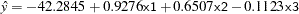Figure 74.3 Diagnostics
Diagnostics
Obs exp Mahalanobis Distance Robust MCD Distance Leverage Standardized
Robust Residual
Outlier
1 e1 2.2536 5.5284 * 1.0995
2 e2 2.3247 5.6374 * -1.1409
3 e3 1.5937 4.1972 * 1.5604
4 e4 1.2719 1.5887   3.0381 *
21 e21 2.1768 3.6573 * -4.5733 *

Figure 74.3 displays outlier and leverage-point diagnostics. Standardized robust residuals are computed based on the estimated parameters. Both the Mahalanobis distance and the robust MCD distance are displayed. Outliers and leverage points, identified with asterisks, are defined by the standardized robust residuals and robust MCD distances that exceed the corresponding cutoff values displayed in the diagnostics summary. Observations 4 and 21 are outliers because their standardized robust residuals exceed the cutoff value in absolute value. The procedure detects 4 observations with high leverage. Leverage points (points with high leverage) with smaller standardized robust residuals than the cutoff value in absolute value are called good leverage points; others are called bad leverage points. Observation 21 is a bad leverage point.

Two particularly useful plots for revealing outliers and leverage points are a scatter plot of the standardized robust residuals against the robust distances (RDPLOT) and a scatter plot of the robust distances against the classical Mahalanobis distances (DDPLOT).

For the stackloss data, the following statements produce the RDPLOT in Figure 74.4 and the DDPLOT in Figure 74.5. The histogram and the normal quantile-quantile plots for the standardized robust residuals are also created with the HISTOGRAM and QQPLOT options in the PROC ROBUSTREG statement. See Figure 74.6 and Figure 74.7.

```   ods graphics on;

proc robustreg data=stack
plots=(rdplot ddplot reshistogram resqqplot);
model y = x1 x2 x3;
run;

ods graphics off;
```

Figure 74.4 RDPLOT for Stackloss Data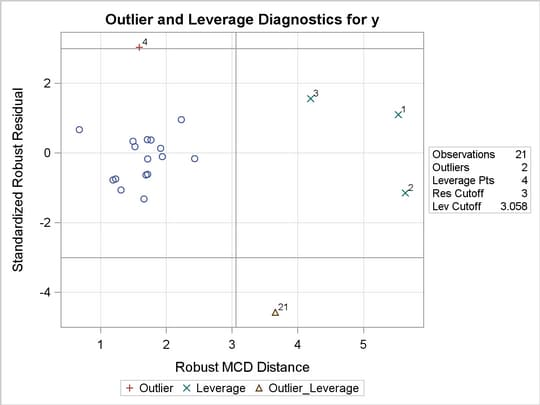Figure 74.5 DDPLOT for Stackloss Data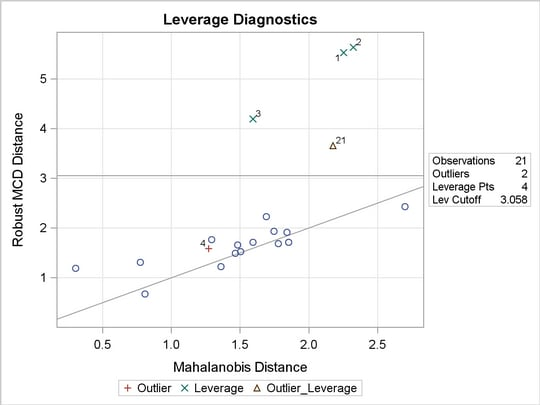Figure 74.6 Histogram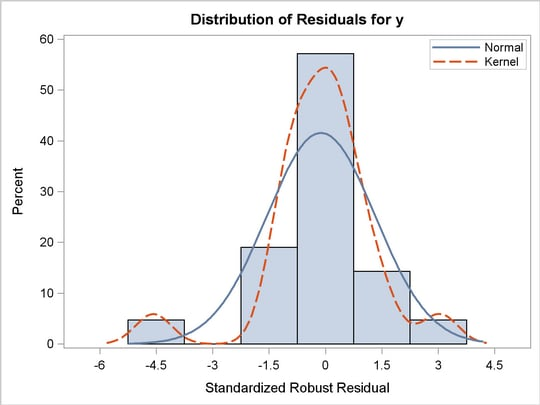Figure 74.7 Q-Q Plot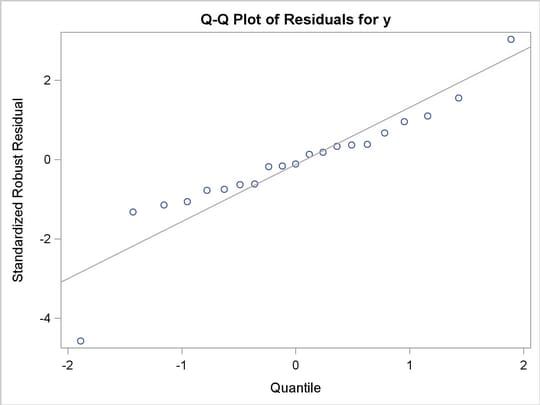These plots are helpful in identifying outliers as well as good and bad high leverage points.

These graphical displays are requested by specifying the ODS GRAPHICS statement and the PLOTS= option in the PROC statement. For general information about ODS Graphics, see Chapter 21, Statistical Graphics Using ODS. For specific information about the graphics available in the ROBUSTREG procedure, see the section ODS Graphics.

Figure 74.8 Goodness-of-Fit Statistics
Goodness-of-Fit
Statistic Value
R-Square 0.6659
AICR 29.5231
BICR 36.3361
Deviance 125.7905

Figure 74.8 displays robust versions of goodness-of-fit statistics for the model. You can use the robust information criteria, AICR and BICR, for model selection and comparison. For both AICR and BICR, the lower the value, the more desirable the model.

Figure 74.9 Test of Significance
Robust Linear Tests
Test
Test Test Statistic Lambda DF Chi-Square Pr > ChiSq
Rho 0.9378 0.7977 1 1.18 0.2782
Rn2 0.8092   1 0.81 0.3683

Figure 74.9 displays the test results requested by the TEST statement. The ROBUSTREG procedure conducts two robust linear tests, the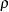test and the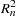test. See the section Linear Tests for information about how the procedure computes test statistics and the correction factor lambda. Due to the large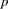-values for both tests, you can conclude that the effect x3 is not significant at the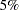level.

For the bisquare weight function, the default tuning constant,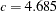, is chosen to yield a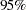asymptotic efficiency of the M estimates with the Gaussian distribution. See the section M Estimation for details. The smaller the constant, the lower the asymptotic efficiency but the sharper the M estimate as an outlier detector. For the stackloss data set, you could consider using a sharper outlier detector.

In the following invocation of the ROBUSTREG procedure, a smaller constant,
, is used. This tuning constant corresponds to an efficiency close to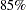. See Chen and Yin (2002) for relations between the tuning constant and asymptotic efficiency of M estimates.

```   proc robustreg method=m(wf=bisquare(c=3.5)) data=stack;
model y = x1 x2 x3 / diagnostics leverage;
id    exp;
test  x3;
run;
```

Figure 74.10 Model Parameter Estimates
The ROBUSTREG Procedure

Parameter Estimates
Parameter DF Estimate Standard Error 95% Confidence Limits Chi-Square Pr > ChiSq
Intercept 1 -37.1076 5.4731 -47.8346 -26.3805 45.97 <.0001
x1 1 0.8191 0.0620 0.6975 0.9407 174.28 <.0001
x2 1 0.5173 0.1693 0.1855 0.8492 9.33 0.0022
x3 1 -0.0728 0.0719 -0.2138 0.0681 1.03 0.3111
Scale 1 1.4265

Figure 74.10 displays the table of robust parameter estimates, standard errors, and confidence limits with the constant.

The refitted linear model is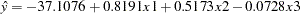Figure 74.11 Diagnostics
Diagnostics
Obs exp Mahalanobis Distance Robust MCD Distance Leverage Standardized
Robust Residual
Outlier
1 e1 2.2536 5.5284 * 4.2719 *
2 e2 2.3247 5.6374 * 0.7158
3 e3 1.5937 4.1972 * 4.4142 *
4 e4 1.2719 1.5887   5.7792 *
21 e21 2.1768 3.6573 * -6.2727 *

Figure 74.11 displays outlier and leverage-point diagnostics with the constant. Besides observations 4 and 21, observations 1 and 3 are also detected as outliers.Previous Page | Next Page | Top of Page